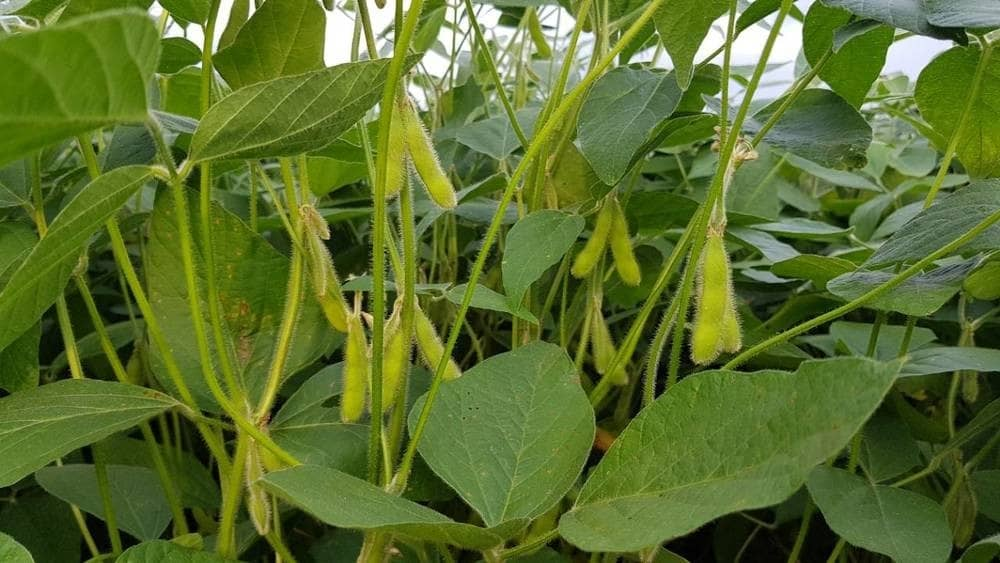# Soybean planting remains at an accelerated pace in Brazil

191Porto Alegre, November 22, 2021 – The planting of the new Brazilian soybean crop had another week of good evolution in most of the country’s producing states between November 13 and 19.

Despite heavy rains having prevented a better advance in the states of Mato Grosso, Goiás, Tocantins, and parts of Minas Gerais, Bahia, and Pará, the pace progressed well at national level, driven by the other states. In Mato Grosso and Mato Grosso do Sul, work is virtually finished. In other states in the Southeast and Midwest, besides Paraná, the last areas to be sown are being completed.

According to a survey carried out by SAFRAS & Mercado, until November 19, Brazilian growers had sowed 84.2% of the total area expected for the country in the 2021/22 season. The percentage is equivalent to approximately 34.098 million hectares out of a total of 40.510 million hectares estimated for planting. In the week earlier, the percentage was 77.6%. In the same period of the previous year, the percentage reached 74.1%, while the five-year average for the period is 77.2%.

In Rio Grande do Sul, 53% of an expected total area of ​​6.33 million hectares were sown, or approximately 3.355 million hectares. In the week earlier, the percentage was 30%. In the same period of the previous year, the percentage was 36%, while the five-year average for the period is 47.6%.

In Paraná, 97% of the area were sown, equivalent to approximately 5.481 million hectares out of total 5.65 million hectares estimated for the state. In the week earlier, the percentage was 92%. In the same period last year, the percentage reached 95%, while the five-year average is 94.2% for the period.

In Mato Grosso, from a total expected area of ​​10.95 million hectares, 99% have already been sown, equivalent to approximately 10.841 million hectares. In the week earlier, the percentage was 99%. In the same period of the previous year, the percentage was 96%. The five-year average for the period is 96.4%.

In Mato Grosso do Sul, the planting is 99% complete of an estimated total area of ​​3.57 million hectares, equivalent to approximately 3.534 million hectares. In the week earlier, the percentage was 96%, while in the same period last year, the percentage was 95%. The five-year average for the period is 95%.

In Goiás, from a total area estimated at 3.88 million hectares, 97% were sown, or nearly 3.764 million hectares. In the week earlier, the percentage was 92%, while in the same period last year, the percentage was 69%. The five-year average for the period is 84%.

In São Paulo, from a total area estimated at 1.22 million hectares, 92% were sown, or approximately 1.122 million hectares. In the week earlier, the percentage was 85%. In the same period of the previous year, the percentage was 50%, while the five-year average for the period is 81.8%.

In Minas Gerais, the percentage sown reaches 85% of a total area estimated at 2 million hectares, the equivalent of approximately 1.7 million hectares. In the week earlier, the percentage was 78%. In the same period last year, the percentage was 86%, while the five-year average for the period is 77%.

In Bahia, from a total area estimated at 1.72 million hectares, 75% were sown, or approximately 1.29 million hectares. In the week earlier, the percentage was 68%, while in the same period last year the percentage was 47%. The five-year average for the period is 46.6%.

Weather forecasts point to another week of large accumulations of rain in the states of Mato Grosso, Goiás, Tocantins, and part of Minas Gerais, Bahia, and Pará between November 19 and 25. The other states should receive some rainfall, but at lower levels.

From November 26 to December 2, the rain must spread across the country, with the highest accumulated levels again being forecast for some states in the Midwest, Southeast, North, and Northeast regions.

SAFRAS Latam